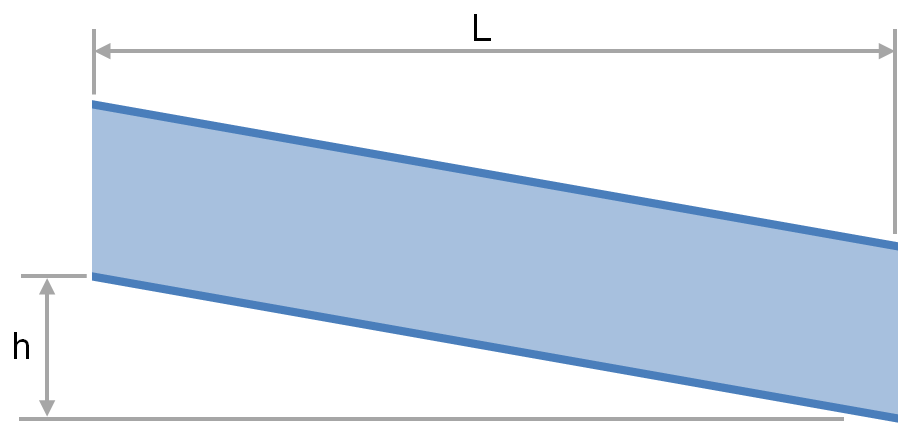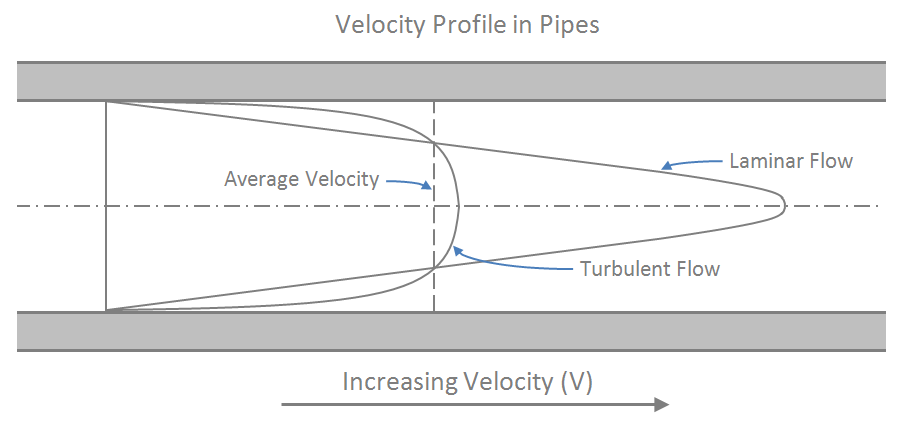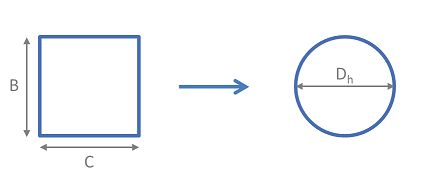f
Neutrium

# Hydraulic Mean Diameter

Flow in Open Channels and Partially Filled PipesThe transport of fluid under gravity is often achieved using partially filled pipes, channels, flumes, ditches and streams. To determine the slope and elevation change required or the flow rate that is achievable one must be able to calculate the head loss and friction factor. This article provides relationships for the calculation of head loss and friction factor for fluids flowing via these conduits.

Reynolds NumberThe Reynolds number is dimensionless and describes the ratio of inertial forces to viscous forces in a flowing fluid. It is used in many fluid flow correlations and is used to describe the boundaries of fluid flow regimes (laminar, transitional and turbulent). This article will show you how to calculate and interpret the Reynolds number.

Hydraulic DiameterHydraulic mean diameter provides a method by which non-circular pipe work and ducting may be treated as circular for the purpose of pressure drop and fluid flow rate calculations. This article provides the equations required to determine the hydraulic diameter for a range of non-circular geometries.